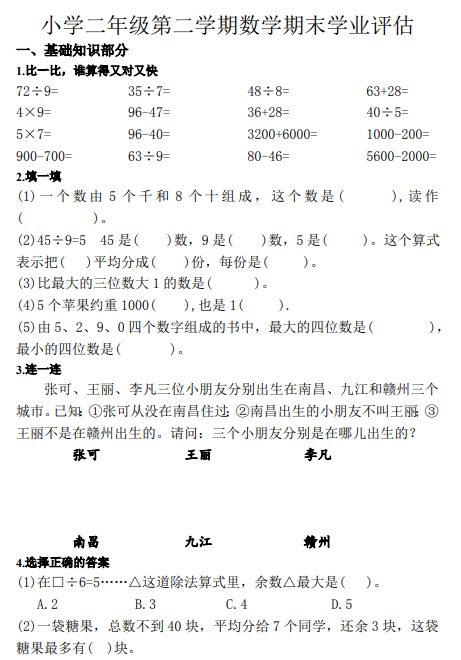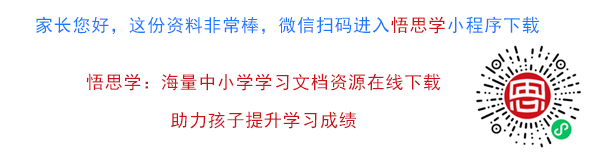1.比一比，谁算得又对又快
72÷9= 35÷7= 48÷8= 63+28=
4×9= 96-47= 36+28= 40÷5=
5×7= 96-40= 3200+6000= 1000-200=
900-700= 63÷9= 80-46= 5600-2000=
2.填一填
(1) 一 个 数 由 5 个 千 和 8 个 十 组 成 ， 这 个 数 是 ( ), 读 作
( )。

【PDF文档3页】最新版人教版小学二年级下册数学期末试题 (9)A4电子版资料_可直接打印_会员免费下载##### 评论信息